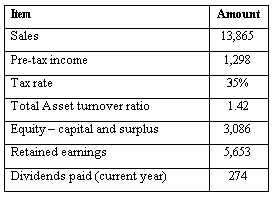### CFA Practice Question

There are 534 practice questions for this study session.

### CFA Practice Question

An analyst has extracted the following information from the financial statements of Myco:What is the net profit margin for Myco?
A. 6.09%
B. 9.36%
C. 14.40%
Explanation: Net profit margin = Net income / Sales = [(1 - Tax rate) x Pre-tax income] / Sales = [0.65 x 1,298] / 13,865 = 0.0609, or 6.09%